# Some pictures in geometric probability

As I discussed in a previous blog post, I have been recently interested in models of randomly growing networks. As a starting point I focused my attention on the preferential attachment rule and its variants, in part because its ubiquity in the literature. However these models miss one key feature, namely the notion of geometry: when a newcomer arrives in the network, she can connect to any vertex, regardless of a possible spatial organization of the network and her current position in that space.

In this blog post I want to discuss some of the most famous (or infamous in some cases) spatial models of network growth. For simplicity, and also to draw pictures, everything will be described in the plane, and specifically on the lattice. These models iteratively build an aggregate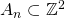as follows: First. Then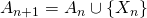where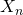is a random point in the boundary of the aggregate, that is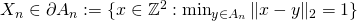. Thus a model is defined by the probability measure one puts on the boundary of the aggregate to select the next point to add.

All the pictures are with half a million particles, and the particles are colored as a function of their age, with blue corresponding to old particles, and red corresponding to young ones.

Eden model

The simplest model uses the uniform measure on the boundary, and it is known as the Eden model. Here is a picture:Theorem: There exists a (deterministic) convex set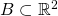such that for any,It is known that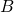is not an Euclidean ball (this should be clear from the picture), though nobody knows whatis exactly. How do you prove such a theorem? Well it turns out to be pretty easy once you have the right machinery. The first step is to realize that the Eden model exactly corresponds to first passage percolation with exponential clocks: imagine that you have i.i.d. exponential random variableson the edges of, and consider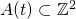to be the set of pointssuch that there exists a path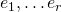from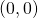towith. In other words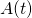is the set of points reached at timeby a fluid released at time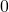inand with travel times on the edges given by the random variables. It is an easy exercise to convince yourself thathas the same distribution asconditionally on(this is actually not true, one in fact needs to put the travel times on the vertices ofrather than on the edges, but let me get away with that small mistake). At this point the continuous version of the above theorem can be rather easily proved via Kingman’s subadditive ergodic theorem, see the Saint Flour lecture notes by Kesten for more details.

Diffusion Limited Aggregation (DLA)

Here we consider the harmonic measure from infinity: pick a point ofuniformly at random from a large circle that contains, and then start a random walk from this point until it hits(which will happen eventually since a simple random walk in two dimensions is recurrent); letbe the latter point. Here is a global picture and a zoom (the same pictures in black and white are provided at the end of this section):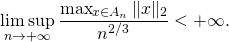A fascinating open problem is to show that for some,which the above picture clearly seems to validate. Note that at the moment nobody can even prove that once rescaled by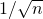the DLA will not converge to an Euclidean ball…Ballistic DLA

Here is a model that, as far as I know, was introduced by Ronen Eldan. Instead of having one particle coming from infinity to hit the aggregate through a random walk, imagine now that the aggregate is constantly bombarded (ballistically). Precisely particles are coming from infinity in every direction (that is on every line) at a constant rate. In other words first select a direction uniformly at random, and then among the lines parallel to that direction that hit the current aggregate choose one uniformly at random. The added point is the boundary point at the intersection of this (oriented) line with the aggregate. Here is a picture where we can see some resemblance with the Eden model:and here is a picture from further away where we can see local resemblance with DLA: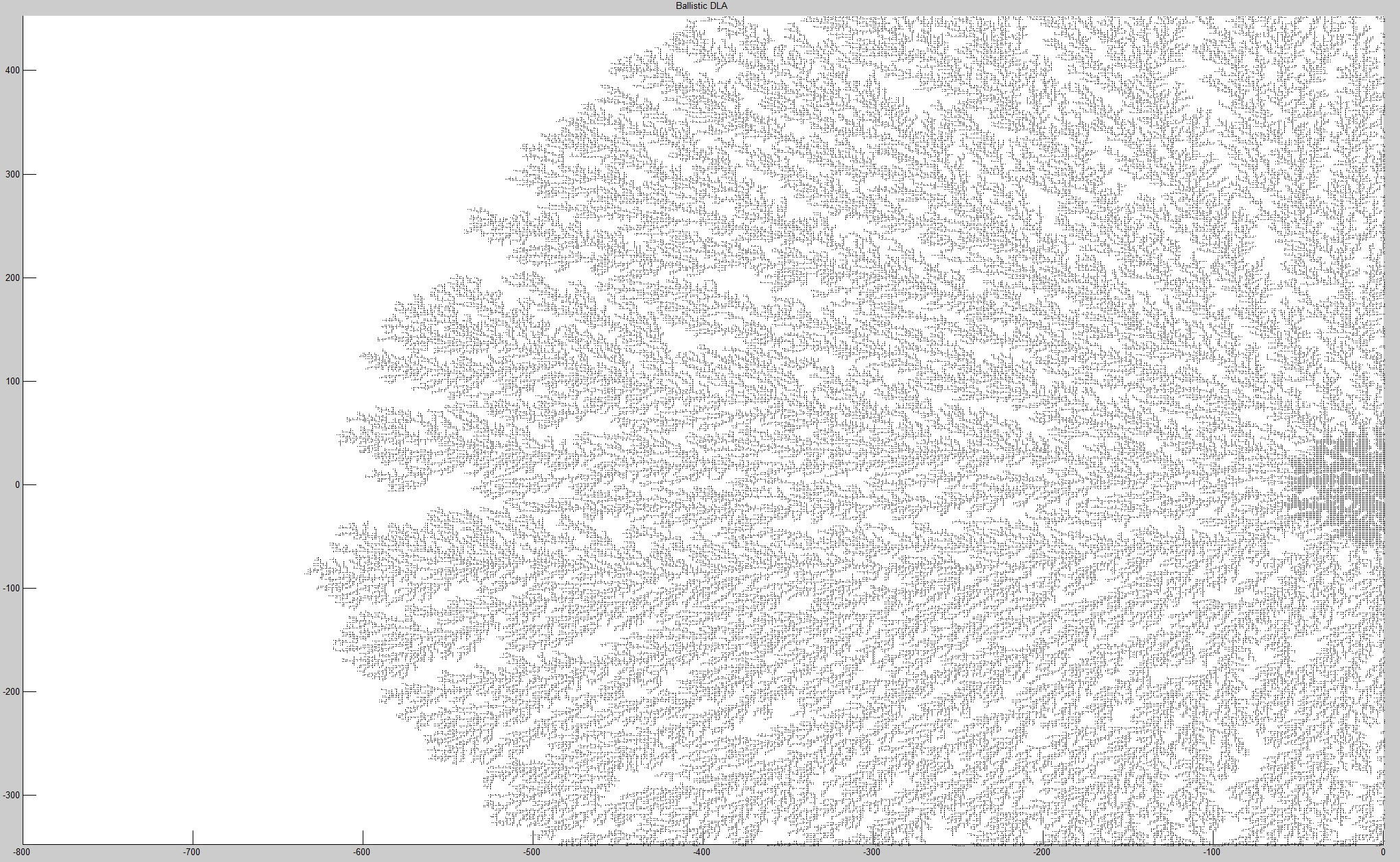Internal Diffusion Limited Aggregation (IDLA)

Finally the IDLA model is yet another modification of DLA, where instead of starting the random walk from infinity one starts it from the origin. Perhaps surprisingly, and on the contrary to everything discussed so far, we know quite a lot about this model! Its limit shape is an actual honest Euclidean ball, see this paper by Lawler, Bramson and Griffeath. In fact we even know that the average fluctuation from the aggregate around its limit is of constant order, see this paper by Jerison, Levine and Sheffield (information about the distribution of these fluctuations is also provided!).

Writing this blog post was quite a bit of fun, and I thank Ronen Eldan, Laura Florescu, Shirshendu Ganguly, and Yuval Peres from whom I learned everything discussed here. To conclude the post here are some intriguing pictures from variants of the above model that I cooked up (unfortunately I’m not sure the models are very interesting though):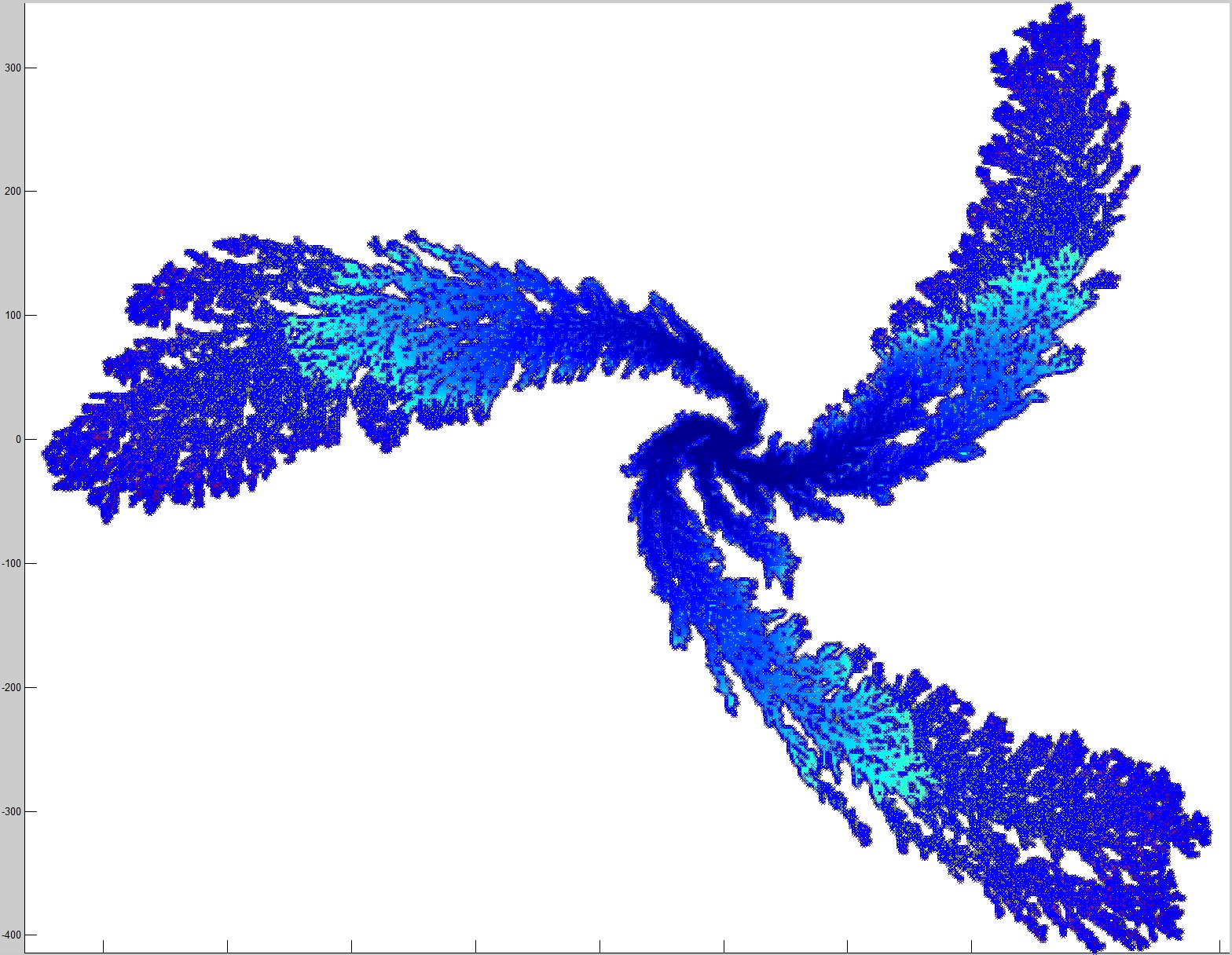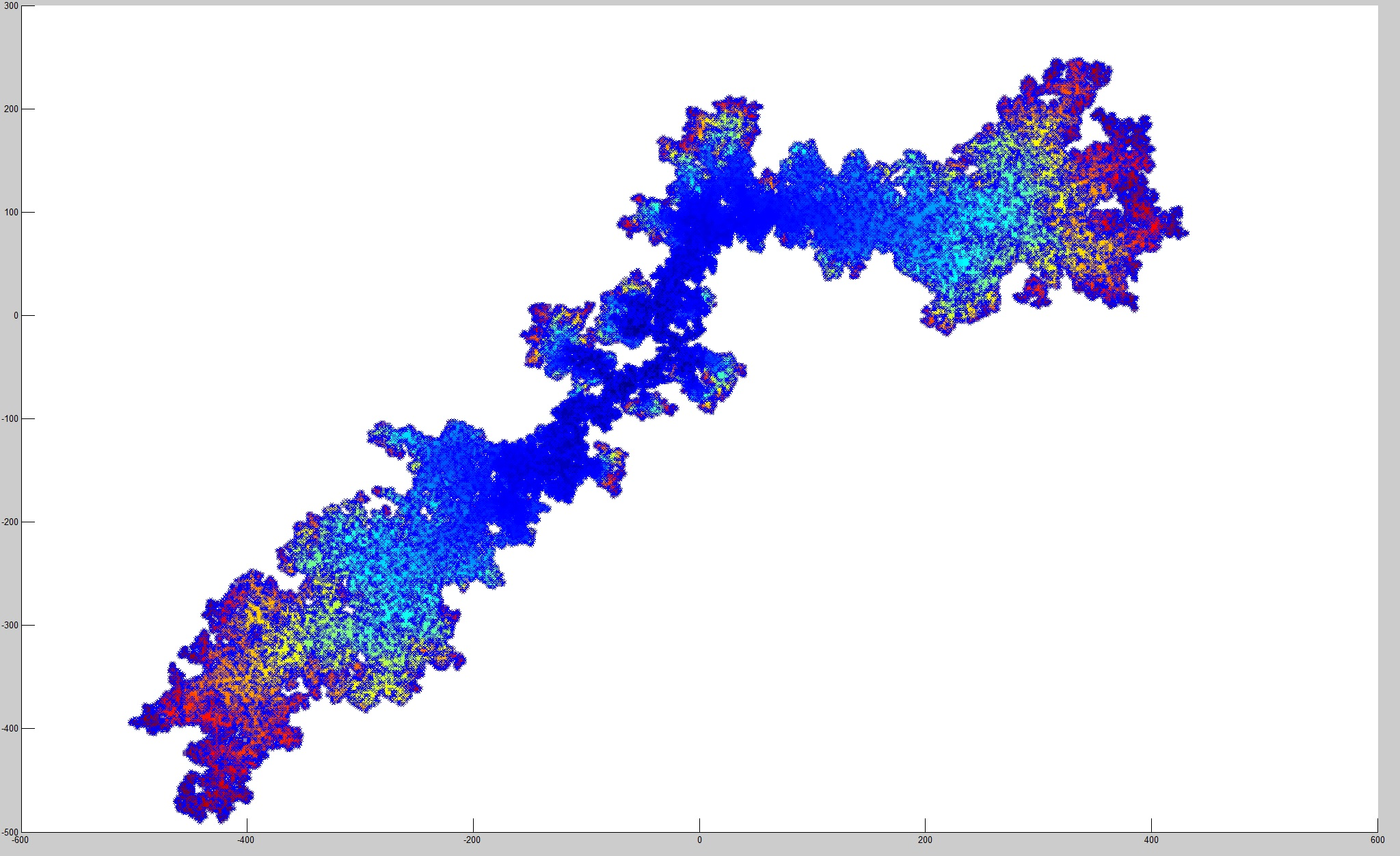This entry was posted in Probability theory, Random graphs. Bookmark the permalink.

## 2 Responses to "Some pictures in geometric probability"

••